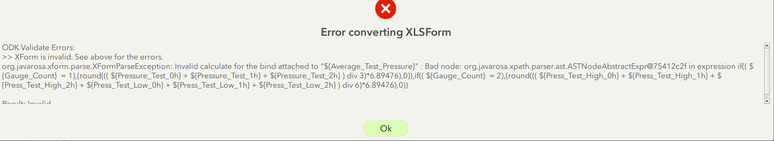# Survey123 - Invalid calculate for the bind in 'if' statement - bad node

1393
4
03-15-2021 05:13 PMby
New Contributor III

Hi, I'm having a lot of trouble getting a calculate statement to work.  I am trying to set a conditional statement for "Average Test Pressure" so that it does the following:

• If there is only one gauge, take 3 readings (0h,1h, and 2 h) and average them, multiply by 6.89476 to get kPa from psi, and round to 0 decimals
• If there are two gauges, take all 6 readings (0h,1h, and 2 h) for both the higher and lower gauge readings, average them, multiply by 6.89476 to get kPa from psi, and round to 0 decimals

However, I keep getting the error that says Invalid calculate for the bind attached to Average Test Pressure: Bad node.I tried playing with the Esri Field Types to make them all double, and the bind type to decimal, but this does not fix my problem.  I need help spotting the problem and fixing it.

I've attached the XLS form.

Any help would be great.

Thanks!

Tags (3)
1 Solution

Accepted SolutionsbyEsri Regular Contributor

Hi @dgray thanks for including your XLSForm, the if() statement is very close! Just need to ensure the parentheses are positioned correctly so that each nested if() is fully enclosed with all three of its arguments, and also that the parentheses around the round() functions are in the right place. Also, an if() statement must contain three arguments: if(condition, value if true, value if false). The 'false' value for the second if() is missing. Your expression could look something like:

if(\${Gauge_Count} = 1, round((((\${Pressure_Test_0h} + \${Pressure_Test_1h} + \${Pressure_Test_2h}) div 3) * 6.89476),0), if(\${Gauge_Count} = 2, round((((\${Press_Test_High_0h} + \${Press_Test_High_1h} + \${Press_Test_High_2h} + \${Press_Test_Low_0h} + \${Press_Test_Low_1h} + \${Press_Test_Low_2h}) div 6)*6.89476),0), 99999))

I've used 99999 for the final 'false' value, but you could replace this with '' for blank.

Hope this helps. Best, Jim

4 RepliesbyEsri Regular Contributor

Hi @dgray thanks for including your XLSForm, the if() statement is very close! Just need to ensure the parentheses are positioned correctly so that each nested if() is fully enclosed with all three of its arguments, and also that the parentheses around the round() functions are in the right place. Also, an if() statement must contain three arguments: if(condition, value if true, value if false). The 'false' value for the second if() is missing. Your expression could look something like:

if(\${Gauge_Count} = 1, round((((\${Pressure_Test_0h} + \${Pressure_Test_1h} + \${Pressure_Test_2h}) div 3) * 6.89476),0), if(\${Gauge_Count} = 2, round((((\${Press_Test_High_0h} + \${Press_Test_High_1h} + \${Press_Test_High_2h} + \${Press_Test_Low_0h} + \${Press_Test_Low_1h} + \${Press_Test_Low_2h}) div 6)*6.89476),0), 99999))

I've used 99999 for the final 'false' value, but you could replace this with '' for blank.

Hope this helps. Best, JimbyEsri Regular Contributor

PS. Noticed the bind::esri:fieldType is set to esriFieldTypeDouble for the integer questions. If you want these to be integer fields in the feature layer, leave the bind::esri:fieldType column blank and the field type will be set by the question type (in this case, esriFieldTypeInteger).by
New Contributor III

Hi Jim,

Thank you for the tip.  I didn't realize you had to provide a false value, so this helped solve my problem.  I found it strange that you did not include a false value for the first if() statement, but it looks like the second if() statement is nested as a false value within the first if() statement, and so only the second if() statement needed an indicated false value.  Is this the case?byEsri Regular Contributor

Hi @dgray yes you're spot on - the second if() is the 'value if false' for the first if(). In other words, if Guage_Count does not equal 1 (condition is false), do this other if() statement. The conditions are evaluated in sequence and returns the first 'true' result, so the 'value if false' for the final if() statement will be the result if none of the conditions are true. Each if() must include three arguments. To illustrate the nesting:

• if(condition, value if true, value if false)
• if(condition, value if true, if(condition, value if true, value if false))
• if(condition, value if true, if(condition, value if true, if(condition, value if true, value if false)))
• ...and so on.

Best, Jim